## Deconstructing the Yield Curve in SQL Server

Oct 13

Written by: Charles Flock
10/13/2016 4:00 PMIn our previous article we took a peek behind the curtain of the SWAPCURVE function gaining insight into the mechanics of turning cash rates, Eurodollar futures prices, and swaps rates into discount factors and continuously compounded zero coupon rates. In this article we attempt to do a little reverse engineering to see if it's possible to take those discount factors and turn them back into the supplied inputs or their associated spot rates.

We will create a table variable to store the data created by the SWAPCURVE function.
DECLARE @SWAPCURVE as TABLE (
mat_date      datetime,
df            float,
rsource       char(1),
zero_cpn      float,
cczero        float
)
The @SWAPCURVE table contains a unique row for each date. Each row identifies the discount factor (df), the continuously compounded zero coupon rate (cczero), and the zero coupon rate (zero_cpn). It is fairly straightforward to convert from one to the other (see the previous blog entry) and for purposes of this article we will only use the discount factors. In addition, the source for each row is identified in the rsource column: cash rates (C), futures (F), swaps (S), or interpolated rates (I). The source of the rates is very important for deconstructing the rates.
Run the following SQL to populate the @SWAPCURVE table.

### Establish the dates

Before doing any calculations, we need to establish the dates that are being used. This curve was generated on 2016-09-09. The spot date is calculated as 2 business days from the processing date. The spot date is essential in the calculation of all maturity dates as they are quoted with respect to the spot date. In talking about a rate for a 10-year swap, for example, the maturity date is calculated as 10 years from the spot date; not 10 years from the processing date.
DECLARE @TODAY as date = CAST('2016-09-09' as datetime)
DECLARE @SPOT as date = wct.BUSINESSDATE(@TODAY,'D',2,'M','')

### Get the discount factor for the spot date

Since cash and swap instruments are quoted with respect to the spot date, their associated discount factors are calculated from the spot date to the maturity date. To obtain the discount factor back to the processing date (the @TODAY variable) we need to, effectively, discount the discount factor for the instrument from the spot date back to @TODAY. This requires that we know the discount factor for the spot date.
DECLARE @df_spot as float =(SELECT df FROM @SWAPCURVE WHERE mat_date = @SPOT)

### A little methodology review

Remember that in calculating the discount factors we use the cash rates until the start of the futures strip, the futures rates until the start of swaps and then we 'bootstrap' the swaps for each coupon date as well as calculating an interpolated swap rate for each coupon date.
At the transition from the cash part of the curve to the futures strip we calculate an interpolated discount factor from the discount factors that surround the start of the futures strip. The discount factor beyond the strip start date is thrown away.
The discount factors from the futures strip are calculated contiguously until the first swap maturity date and any after that date are thrown away. An interpolated discount factor is calculated for each swap coupon date prior to the first swap maturity date.

### Recovering the swap rates

In many ways it is simpler to work backward from the end of the curve to the beginning of the curve. Remember that the swaps part the curve relied on a bootstrapping process which used this formula: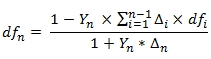Since we know all of the discount factors we can reconstruct the swap part of the curve using this formula: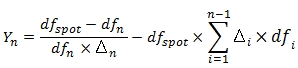The following SQL implements this formula and puts the results into the #swaps temporary table. We use the BONDCF table-valued function and BUSINESSDATE scalar function to calculate all the coupon dates associated with the swaps so that we pick up the correct deltas and discount factors. We use the YEARFRAC function to calculate the deltas.
--Recover the swap rates
SELECT
*,
ROUND((@df_spot-df)/((df*delta)-(@df_spot*-LAG(bootstrap,1,NULL) OVER (ORDER BY q.mat_date))),10) as [Swap Rate]
INTO
#swaps
FROM (
SELECT
*,
SUM(delta * df) OVER (ORDER BY p.mat_date) as bootstrap
FROM (
SELECT
n.mat_date,
S.rsource,
S.df,
wct.YEARFRAC(@SPOT,n.mat_date,2) - wct.YEARFRAC(@SPOT,LAG(n.mat_date,1,@SPOT) OVER (ORDER BY n.mat_date),2) as delta
FROM (
SELECT
FROM
(SELECT max(mat_date) as maxDate FROM @SWAPCURVE)n(mdate)
CROSS APPLY
wct.BONDCF(@SPOT,n.mdate,0,0,NULL,2,NULL,NULL,NULL,NULL)k
)n
INNER JOIN
@SWAPCURVE S
ON
n.mat_date = S.mat_date
WHERE
n.mat_date > @SPOT
)p
)q
The #swaps table should contain the following results

### Recovering the futures strip

We can use the following formula to calculate the rates associated with the futures contracts.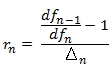We need to calculate the futures strip start date to establish a starting point for the calculation. For yield curve construction we know that the Eurodollar futures contracts are delivered on the 3rd Wednesday of the month and that the strip is in 3-month increments. By using the FIRSTWEEKDAY and EDATE function to determine the strip start date, it becomes quite straightforward to calculate the associated rates using the formula. The following SQL puts the results in the #futures temporary table.
--Recover the futures rates
SELECT
*
,ROUND(((LAG(df,1,NULL) OVER (ORDER BY mat_date)/df)-1)/wct.YEARFRAC(LAG(mat_date,1,NULL) OVER (ORDER BY mat_date),mat_date,2),10) as [Futures Rate]
INTO
#futures
FROM (
SELECT
mat_date,
df,
rsource
FROM (
SELECT
--Figure out what the start date is for the futures strip
FROM
@SWAPCURVE
WHERE
rsource = 'F')n(date_start)
INNER JOIN
@SWAPCURVE S
ON
n.date_start = S.mat_date
UNION ALL
SELECT
mat_date,
df,
rsource
FROM
@SWAPCURVE S
WHERE
rsource = 'F'
)n
This is what the #futures table should look like.

### Recovering the cash rates

We can use the following formula to recover the cash rates.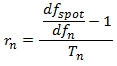Tn in this formulation is the time, in years, from the spot date to the maturity date of the cash instrument. The following SQL uses the YEARFRAC function to calculate the time values in implementing the formula and puts the results in the #cash temporary table.
--Recover the cash rates
SELECT
mat_date,
df,
rsource,
ROUND((@df_spot/df - 1) / wct.YEARFRAC(@SPOT,mat_date,2), 10) as [Cash Rates]
INTO
#cash
FROM
@SWAPCURVE
WHERE
rsource = 'C'
AND mat_date > @SPOT
This what the #cash table should look like.
Notice that in our previous SQL we excluded all the maturity dates less than or equal to @SPOT. If the maturity data is less than or equal to the spot date, then the calculation of the rate value does not have to be adjusted by the discount factor for the spot date. It requires a different formulation.
The calculation for the tom/next maturity date (the first entry in the @SWAPCURVE table) is straightforward. This SQL adds the tom/next rate to the #cash temporary table.
INSERT INTO
#cash
SELECT
mat_date,
df,
rsource,
ROUND(((1/df)-1)/wct.YEARFRAC(@TODAY,mat_date,2),10)
FROM
@SWAPCURVE
WHERE
mat_date < @SPOT
The calculation for the spot rate is similarly straightforward but relies on the tom/next rate calculated in the previous step. This SQL adds the spot rate to the #cash temporary table.
INSERT INTO
#cash
SELECT
@SPOT,
S.df,
S.rsource,
ROUND(((n.df/s.df)-1)/wct.YEARFRAC(n.mat_date,@SPOT,2),10)
FROM
(SELECT TOP 1 mat_date, df FROM #cash ORDER BY mat_date asc)n
INNER JOIN
@SWAPCURVE S
ON
S.mat_date = @SPOT

### Are we done yet?

Not quite. We still need to account for the cash rate that was used for the interpolated rate for the start of the futures strip. Recall that the strip start date was 2016-12-21. The interpolated rate for the strip start date was calculated using the cash rates. We have already calculated the cash rates out to 3 months. Given that we know the interpolated discount factor for the strip start date and the discount factor for the 3-month cash rate, can we infer the 6-month cash rate?
When we transition from the cash part of the curve to the futures part of the curve we use discount factor interpolation (see the DFINTERP function) to calculate the discount factor for the strip start date. The formula for discount factor interpolation is: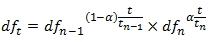Where t is time in years and tn-1 ≤ t ≤ tn and a = (t1-t) / (t2 – t1). Simple re-arrangement of terms gives us the following equation.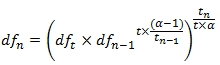The following SQL implements the re-arranged equation and stores the result in the #cash temporary table. We use the TENOR2DATE function to calculate the maturity dates, the FIRSTWEEKDAY and EDATE functions to calculate the strip start date and the YEARFRAC function to calculate all the time values.
--Calculating the cash rate used to start the futures strip
DECLARE @date_3m as datetime = wct.TENOR2DATE('3M',@TODAY,@SPOT,'')
DECLARE @date_6m as datetime = wct.TENOR2DATE('6M',@TODAY,@SPOT,'')
DECLARE @date_futures_start as datetime =(SELECT DATEADD(d,14,wct.FIRSTWEEKDAY(wct.EDATE(MIN(mat_date),-3), 4)) FROM @SWAPCURVE WHERE rsource = 'F')
DECLARE @t as float = wct.YEARFRAC(@TODAY,@date_futures_start,3)
DECLARE @tnminus1 as float = wct.YEARFRAC(@TODAY,@date_3m,3)
DECLARE @tn as float = wct.YEARFRAC(@TODAY,@date_6m,3)
DECLARE @alpha as float =(@t-@tnminus1)/(@tn-@tnminus1)
DECLARE @dft as float =(SELECT df FROM @SWAPCURVE WHERE mat_date = @date_futures_start)
DECLARE @dfnminus1 as float =(SELECT df FROM @SWAPCURVE WHERE mat_date = @date_3m)
DECLARE @dfn as float = POWER(@dft*POWER(@dfnminus1,@t*(@alpha-1)/@tnminus1),@tn/(@t*@alpha))

INSERT INTO
#cash
SELECT
@date_6m,
@dfn,
'C',
ROUND(((@df_spot / @dfn) - 1) / wct.YEARFRAC(@SPOT,@date_6m,2), 10)
It's worth noting that the 6-month cash maturity date coincides with the first coupon date from the swap part of the curve. However, other than in the interpolation of the discount factor for the start of the futures strip, this rate is not used. The 6-month rate used in the bootstrapping process is calculated using discount factor interpolation and the futures discount factors.

### Producing the results

The cash part of the curve:
SELECT
mat_date,
[Cash Rates]
FROM
#cash
ORDER BY
mat_date
This produces the following result.
The futures part of the curve:
SELECT
100 *(1-[Futures Rate]) as [Futures Price]
FROM
#futures
WHERE
rsource <> 'I'
This produces the following result.
The swaps part of the curve:
SELECT
mat_date,
[Swap Rate]
FROM
#swaps
WHERE
rsource <> 'I'
This produces the following result.

### Calculating the benchmark spot rates

The benchmark spot rate or zero-coupon rates can be calculated from the discount factors. When we talk about spot rates in this context we are not talking about the cash rate for the spot date (which we used in many of our calculations) but rather the yield on a zero coupon bond implied by the discount factors. This calculation should use the same compounding assumption as the swaps curve, which in our example is semi-annually.
SELECT
mat_date,
df,
[Swap Rate],
ROUND(2*(POWER(@df_spot/df,1e+00/(2*DATEDIFF(year,@SPOT,mat_date)))-1),10) as [Benchmark Spot Rate]
FROM
#SWAPS
WHERE
rsource = 'S'
This produces the following result.

### Conclusions

By deconstructing the swap curve we have gained more insight into how cash rates, swap rates, and futures prices are converted into discount factors and continuously compounded zero coupon rates. This article has hopefully helped in understanding the link between the inputs and the outputs to those calculations. To find out more about how to build the yield curve, see our article on Constructing a Yield Curve in SQL Server.

If you are interested in taking advantage of the powerful tools in the XLeratorDB library of functions, you should download the free 15-day free trial today.

If you are not a SQL Server user you should try out the XLeratorDLL library of functions, which also has a free 15-day trial. Download that and see how easy it is to incorporate this functionality into your .NET code.

Got questions? Send us an e-mail at support@westclintech.com or directly to me at cflock@westclintech.com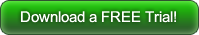Tags:
Categories:
Location: BlogsThe WestClinTech Blog

## Search Blogs

 KeywordsPhrase

## Blog Archives

Archive
 < July 2021 >
SunMonTueWedThuFriSat
27282930123
45678910
11121314151617
18192021222324
25262728293031
1234567
Monthly
Go

### SupportCopyright 2008-2021 Westclintech LLC         Privacy Policy        Terms of Service